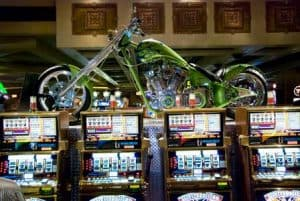# Binomial probability.Binomial probability allows us to show that if we play roulette, except on rare occasions with luck, the bank always wins.

Have you been to Las Vegas?. It’s an interesting city to visit. Once. Two, tops. Casinos are an amazing thing, with everyone playing like crazy with the hope of getting rich with little effort.

But, who do you think that pays everything you see in Las Vegas?. You get it, those who gamble. The banker never loses. Take my advice and don’t risk your money in casinos, because the likelihood of winning is rather scarce and, even in you win, the more likely is that you don’t win too much money. Of course, this may not be true if you bet large quantities of money, but if you have so much money you will have no need to bet to get rich.

Let’s see with an example how difficult is to become a millionaire by this method. Think about one of the possible gambles you can do with roulette: the street bet or three-number bet. For those of you who have not played ever, our wheel has 38 numbers.

## Let’s play roulette

In this bet, we put our gaming chips on three numbers of the same row and the wheel spins. Suppose we bet one euro on every play. The street bet pays 11 to one, which means that if the ball lands on any of our three numbers the banker gives us back our euro and 11 more. But if the ball lands in any other of the 38 numbers, we’ll lose one euro.

So the likelihood of winning is p = 3/38 and of losing is q = 35/38. Let’s consider first what the theoretical net gain of each play is: it is the sum of 11 times the probability of winning minus the probability of losing one euro:

Net gain = (3/38 x 11) – 35/38 = -0.0526 €

This means that, on average, we’ll lose something more than five cents on every play. And what if we play 300 times in a row? Can we get rich then?.

Then neither, because the expected gain is the average profit of each play by the total number of bets, i.e. -0.0526 x 300 = €-15.78. So why on earth do people gamble if the more times you bet the higher your expected loss?. Precisely because it’s an expected amount, but the number of times you can win or lose follows a binomial distribution, so there will be lucky people with small loses or even profits, but also unfortunate ones who will lose much more than expected.

The next point that you may be thinking about is what our chances of winning are if we play three hundred times in a row. Let’s find it out.

Let’s call W the number of times we win and G our net profit after 300 bets. The net gain is the number of times you win multiply by 11 (remember that the bet is paid eleven to one) minus the number of times you don’t win (and lose one euro). On the other hand, the number of times you lose equals the number of bets minus the number of times you win. Thus:

G = 11 W + (-1)(300 – W) -> 12 W – 300

If we win, our net gain G must be greater than zero. If we put it in the above equation:

12W – 300 > 0

We have

W > 300/12

W > 25

This means that, to avoid losing money, we have to win a minimum of 25 times out of the 300 we play. And 25 are many or few times?. To me, honestly, it seems a lot, but we can calculate the probability of winning 25 times.

## Binomial probability

We have already said that the model of the wheel follows a binominal probability frequency distribution:

Where n is the number of bets, k is the number of successes, p the likelihood of winning and q or (1-p) the probability of losing. If we substitute the letters of the equation for our data (n=300, p=3/38 and k=25) we can calculate what is the probability of winning at least 25 times. The problem is that numbers become so large that it becomes difficult to deal with, so I would advise you to use a statistical program or any available Internet calculator to do it. I’ve done it and I’ve came up with a probability of 42%.

## The house always wins

Good!, someone of you may think. A 42% chance of winning is not so bad. But think for a moment that what is not bad, at least from the casino’s point of view, is the 58% chance of losing we have. And besides, 42% is the overall probability of winning. If you calculate the number of bets you have to win to get a net gain of 100 euros, you will see that it is more than 34, and the probability of winning more than 34 bets out of 300 goes down to 2.2%.